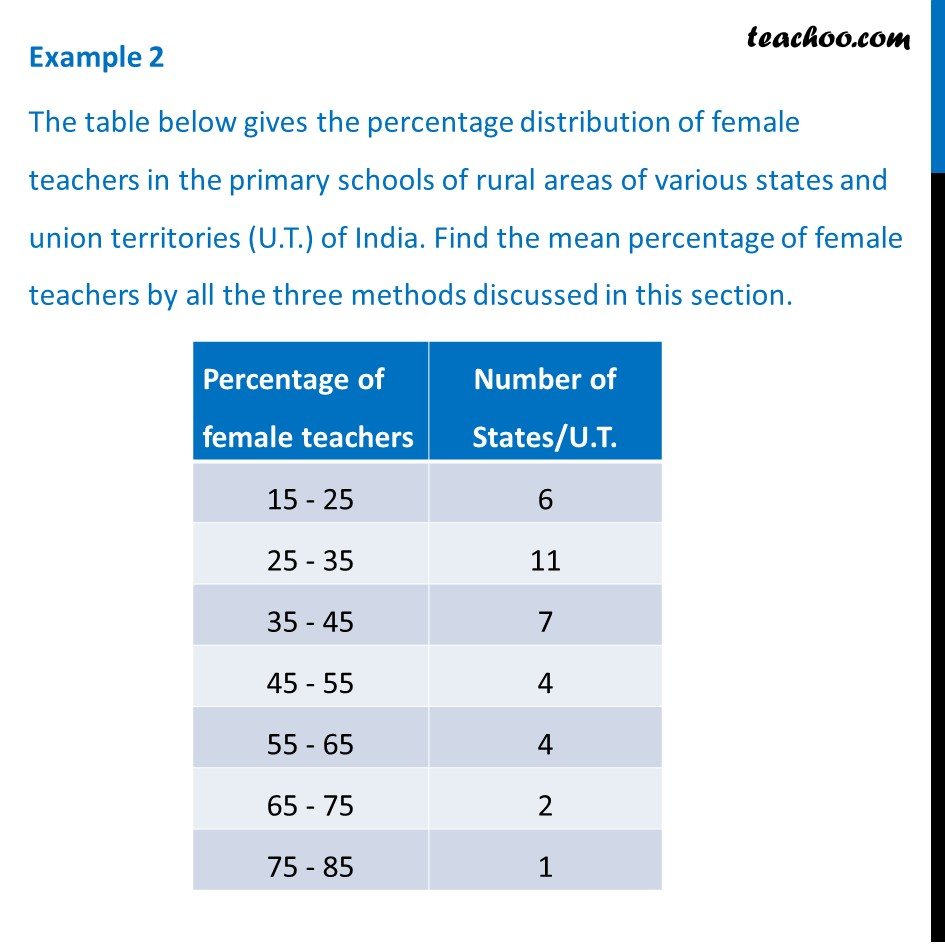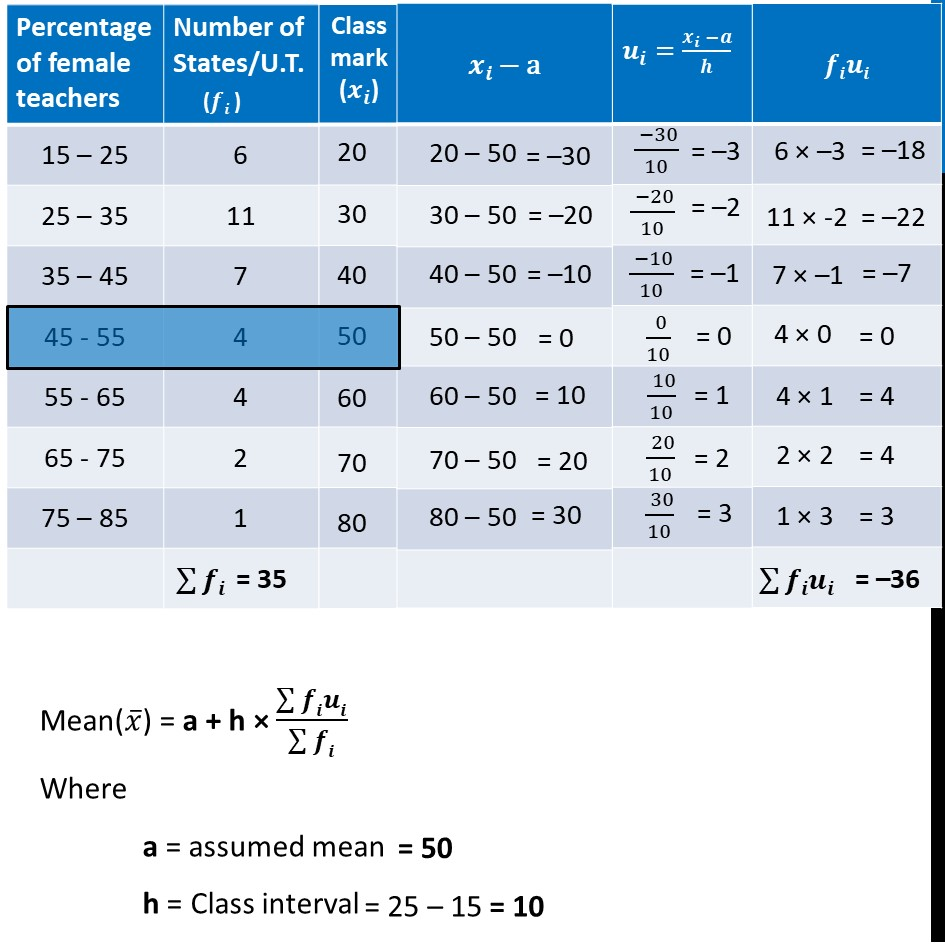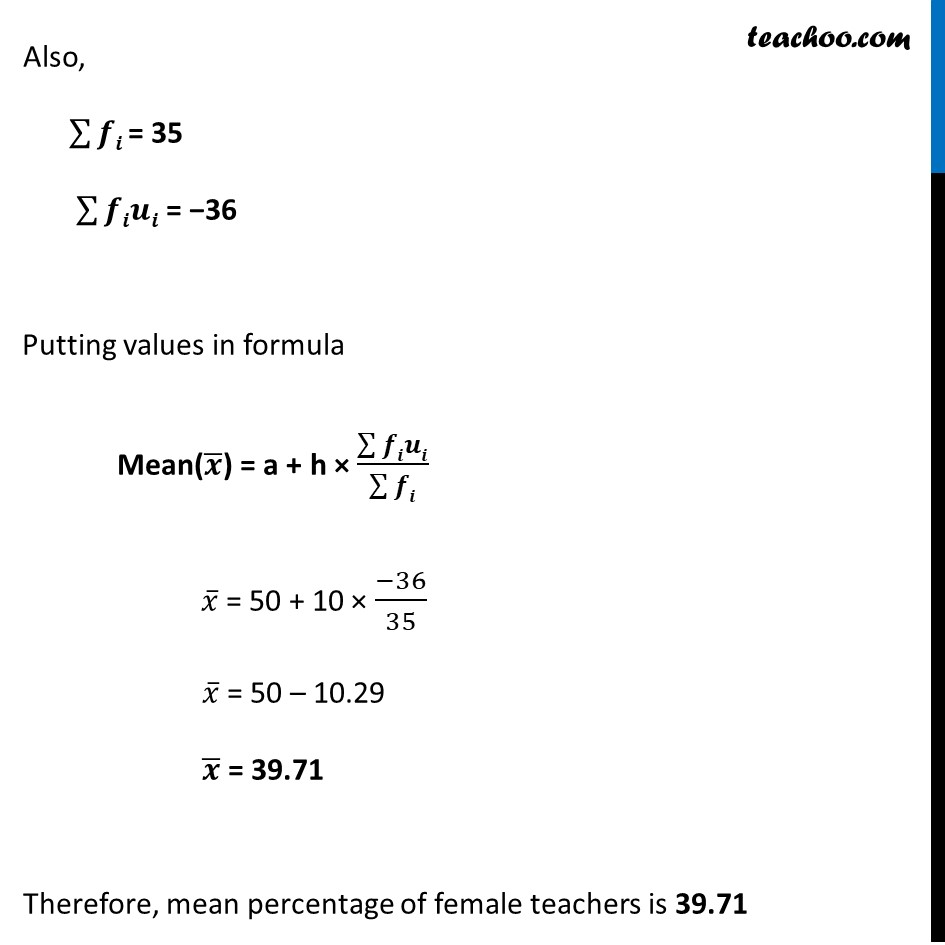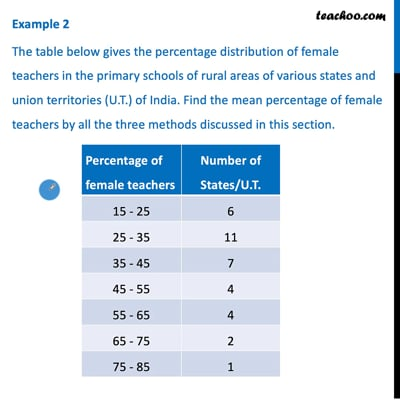Examples

Chapter 14 Class 10 Statistics
Serial order wiseThis video is only available for Teachoo black users

Solve all your doubts with Teachoo Black (new monthly pack available now!)

### Transcript

Example 2 The table below gives the percentage distribution of female teachers in the primary schools of rural areas of various states and union territories (U.T.) of India. Find the mean percentage of female teachers by all the three methods discussed in this section. Mean(𝑥 ̅) = a + h × (∑▒𝒇𝒊𝒖𝒊)/(∑▒𝒇𝒊) Where a = assumed mean h = Class interval Also, ∑▒𝒇𝒊 = 35 ∑▒𝒇𝒊𝒖𝒊 = −36 Putting values in formula Mean(𝒙 ̅) = a + h × (∑▒𝒇𝒊𝒖𝒊)/(∑▒𝒇𝒊) 𝑥 ̅ = 50 + 10 × (−36)/35 𝑥 ̅ = 50 – 10.29 𝒙 ̅ = 39.71 Therefore, mean percentage of female teachers is 39.71